﻿ Using Residual Analysis to Validate Watermelon Date of Planting and Plant Spacings Experiment ModelsPublications are Open
Access in this journal
Article Versions
Export Article
• Normal Style
• MLA Style
• APA Style
• Chicago Style
Research Article
Open Access Peer-reviewed

### Using Residual Analysis to Validate Watermelon Date of Planting and Plant Spacings Experiment Models

I.U. OBI, OKEKE G.C. , OSELEBE H.O., T. VANGE
Applied Ecology and Environmental Sciences. 2017, 5(2), 49-59. DOI: 10.12691/aees-5-2-4
Published online: November 01, 2017

### Abstract

Picking a model for a problem is a strong task. If the model fits well then it can be used to increase the understanding and learning of the problem and/or for prediction. Several procedures and steps have been adopted by researchers for dates of planting and plant spacing studies to suit their objectives. The ever-proliferation of Statistical steps available to researchers has given room for use of different statistical design for research into finding optimum date of planting and plant spacing for crops. Considering the subtle differences, advantages and disadvantage that these statistical designs brings, the results of such analysis may lead to false conclusion or be less reliable at least for comparative purposes. There is need to look at plant spacing trials again to see the possibility of proffering a statistical model that could be commonly used by researchers. The main purpose of this work is to apply the residual analysis to check the suitability of the series of similar experimental model to describe the effects of date of planting and plant spacings on yield of watermelon with the view of predicting optimum date of planting and plant spacing. Results show that the series of similar experiment methodology is able to model the changes associated with different date of planting and plant spacings. The questions associated with model adequacy were discussed.

### 1. Introduction

Studies on plant spacing have been conducted extensively for several crops across different ecological zones. A review of studies on watermelon plant spacings indicates that these research were necessitated by environmental pattern 1, 2; pest management 3, 4, 5, 6, 7, disease management 4, 8; and fruit yield 9.

Several statistical procedures and steps have been adopted by researchers for plant spacing studies to suit their objectives. Obi 10 proposed series of similar experiment models for plant spacing experiments. Some researchers 11, 12, 13, 14, used this series of similar experiment model in their studies. A survey of other works on plant spacing show that 15 used a randomized block design with four complete blocks to study the effect of spacings and residue rate effect on growth partitioning and yield of egusi-melon. Also 4, 8 used randomized complete block design in their experimental trials, while 16, 17 in their trial on response of soybean lines with juvenile trait to day length and plant spacing combined both greenhouse and field work. In both cases they used factorial arrangement. Acikgoz 18 in his studies on effect of plant spacing and plant method on rice yield per day also used factorial experiment. Fakorede 19, in maize planting date trial, used a randomized complete block design (RCBD) with a split-plot arrangement. He assigned dates to main plot, plant spacing to sub plot and genotypes to the sub-sub plot. Chand and Singh 3, used split-plot design in their study of effect of plant spacing on Stem Rot (SR) incidence in rice, 20 also used split-plot design for his studies. Both 3 and 20 assigned spacing to sub plot and variety to sub-sub plot. Obi 12, in rice planting dates used residual analysis to validate rice sowing date experiment model.

Federer Design: Walter T. Federer, Professor of Biological Statistics, Biometrics Unit, College of Agriculture and Life Sciences, Cornell University, Ithaca, New York (2001) (Personal communication with Obi, I.U.) gave his premise for Time (Date) planting of planting which is stated as follow: “Variety x Location, Variety x Year, and Variety x Location x Year interactions are mostly Date of Planting x Variety interaction”. Federer said, “if I were setting up an experiment on Date of Planting, I would use a number of locations (Different), and Years and in each Year and Location, I would have a replicated experiment where the replicates were Date of Planting, say three (3) Dates with two (2) replicates, each, Whole-plots would be crops and the Split-plots would be Varieties within Crops”. Some form of an Analysis of Variance (ANOVA) as suggested by Federer is as presented in Table 1. Obi communication, thinks that what these researchers are doing is “fitting data to a model” not “fitting model to data.” He did this by illustrating the field layout of those designs derived by those researchers.

1.1. Verifying the Adequacy of the Linear Model

The outlier tests 9, 17, 21, 22 as well as the variance homogeneity tests 14, 23, 24 have been used. Kirton, personal communication with Federer 25, department of Agriculture, New South Wales, Sydney, Australia, as cited by 26, suggested that after one has a “correct model,” one should as follows in searching for a discrepant “treatment” or “block”: (i) compute estimate residuals, (ii) use absolute values of the residuals , and (iii) perform a standard analysis of variance (ANOVA), the same one as used for the response, and/or multiply comparisons on the absolute values of the estimated residuals. If the model is “correct”, then the null hypothesis should be true for all the categories in the ANOVA except for residuals of the absolute values of residuals. That is, the expected value for each F-test is “one”. If the null hypothesis (hypotheses) is/are not true, then this procedure can be used to pinpoint discrepant treatments, blocks, etc., in the experiment 25.

Federer et al., 27 did “Studies of Residual Analysis” titled; “Analysis of Absolute Values of Residuals to test Distributional Assumptions of Linear Models for Balanced Designs”. Although statistical models are not largely used in plant spacing data analysis, a verification of the suitability of the model is not always checked. Hence, indicating that the search for the Model for plant spacing experiment is continuing and the Model and Field Layout are yet to be confirmed.

The main purpose of this work is to apply the residual analysis to check the suitability of the conventional series of similar experiment proposed by 10, 28 to describe the effect of plant spacings on watermelon cultivars. Additionally, the authors expect that this paper is capable of introducing a step-by-step procedure for the implementation of the residual analysis to any statistically significant model. Also, answers would be proffered to questions concerning model validity such as:-

(i) How can I tell if a model fits my data?

(ii) How can I assess the sufficiency of the functional part of the model?

(iii)How can I test whether or not the random errors are distributed normally?

1.2. Statistical Model

A statistical model is a mathematical model which contains error with a specific probability distribution. Usually, this model is used to predict the value of one of the variables when the other is known, under specific conditions 29. In a statistical model, two or more variables are related using regression analysis equations. These equations are mainly used to predict the dependent variable, Y, as a function of the independent variable, X. In the analysis, some assumptions are necessary 30, 31.

The dependent variable, X, is considered free of errors because X is not a random variable. There is a linear relationship between Y and X and the statistical model that relates Yi to Xi as given by: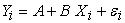(1)

For i = 1, . . ., n, where n is the number of observations.

In equation 1, A and B are unknown constants to be estimated and they are called parameters of the regression model. The random value, εi is the denominated random error. The value of εi for any observation will depend on both a possible error of measurement and other variables different from Xi that were not measured that could affect Yi. The values of εi for any observation will depend on both a possible error of measurement and other variables different from Xi that were not measured that could affect Yi. The values of εi are random variables, assuming the following assumptions:

(i) The average of εi values is equal to zero and its variance, is unknown and constant for 1 ≤ I ≤ n;

(ii) εi values are not correlated;

(iii) The distribution of εi values is normal for 1 ≤ I ≤ n.

Second and third assumptions imply that εi values are mutually independent. The regression line is, in general, unknown and therefore must be estimated through the sampling data. In the particular case where the regression of Y in relation to X is linear, the best fit line can be written as: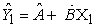(2)

Where the symbol “caret” (^) denotes estimate (estimator) Â and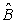are determined by the least squares method and Ŷ1 are the estimated values of Y1 using Equation 2 such that the differences between Y1 and Ŷ1 shall be minimum. Generally, these differences are known as residuals, i.e., errors associated to the predicted values of Y1 corresponding to each X1 value and which can be calculated through the following expression: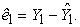(3)

A discussion of a simple linear model considering two variables X and Y would enhance our understanding of procedures used to examine the adequacy of a model.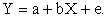The variable e denotes random error, that is, if there were no error, Y would be a deterministic linear function of X.

When is a model good? At first, one might say when there is no error. But for all the data that we consider in this class there will always be error. Actually we will say a model is good if there is no connection between e and a +bX: that is, the random error is free of X. hence, for predicting Y, we have found the model that contains all the information based on X. Now there may be other variables which help in predicting Y. These will be contained in e.

Model Assumption: So the assumption we want to verify on a model is: the random error component is independent of the X component. How would we check this assumption? If we knew the random errors, e, we would just plot them against a + bX. A random scatter plot would indicate that the errors do not depend on a a + bX; i.e., the errors are free of a + bX. Thus the model is good. However, we don’t know the errors, we only know Y and X. But using Y and X we estimate a and b. This leads to an estimate of a + bX, the predicted value of Y, which we label as Ŷ. Our estimate of the error is Y – Ŷ. This is called the residual, literally, what’s left. We will denote the residual by ê, that is, ê = Y – (â +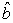X). Then we can check our model assumption by plotting ê versus Ŷ. This is called the residual plot. A random scatter indicates a good model. If it not a random scatter then we need to rethink the model 28. The verification of residuals normality can also be analyzed by plots, such as normal score and normal probability graphs. In these graphs, the assumption of normality is valid if the points in the graph are localized approximately along a straight line. However, in case of doubt, the linearity can be confirmed using a statistical test of normality, such as the one proposed by 29.

### 2. Experimental Procedures for the Time of Planting Experiment

The research was carried out at the Teaching and Research Farm of the Department of Crop Production and Landscape Management, Ebonyi State University, Abakaliki. The study region falls within the tropical rain forest zone of South-Eastern Nigeria and it is located between latitude 0604IN and longitude 080 65IE at the elevation of 71.44mm above sea level. It has a bimodal rainfall pattern. It’s rainfall per annum ranges between 1700-2000mm in April to July and August to November for early and late season plantings, respectively. The relative humidity at dry season ranges between 60-80% 32 and the soil belongs to the order ultisols.

The experimental plot was ploughed and harrowed using a tractor. Seed beds were prepared in an area of 0.553ha.The land area measured 0.553ha. The designated plant distances were measured and marked out in their respective plots and seeds were hand-sown at the rate of two (2) seeds per hole on the bed. Since they were nine (9) treatment combinations, each block was divided into nine (9) plots, each measuring 3.6m x 1.4m. Spacing between plots and blocks was one meter (1m). The nine treatment combinations were randomly assigned to the experimental units. The three (3) varieties of watermelon were taken to the field for evaluation trial. The evaluation plots were laid out in a randomized complete block design (RCBD) with three replications. Four experiments were laid out as series of similar experiments representing the four planting dates. Each experiment consists of three varieties of watermelon (Sugarbaby, Kaolack and F1 Koloss) and three levels or rates of poultry manure (0, 5 and 10t/ha) each four planting date corresponding to a 3 x 3 factorial experiment in randomized complete block design (RCBD) of three blocks or replications. The experiment comprised four experiments which were laid out as series of similar experiments 12 representing the four planting dates using 1.0m x 0.6m plant spacing. The first experiment commenced on April 4, 2014 and the subsequent experiments followed after every two weeks, that is, April 18, May 2 and 16, 2014.

• Figure 1. The field layout for Date (Time) of Planting Experiment as Series of Similar Experiments (d1 = Fisrt Date of Plant and d4 = Date of Planting 8 weeks after)

Data on yield was collected at maturity. Fruit yield per plot was converted to tones per hectare. The Analysis of Variance was performed using the procedure outlined by 33, 34 for each measured parameter. Means that had a significant F-test were separated using LSD(0.05) 26, 35. The data analysis was carried out in stages.

### 3. Test for Homogeneity of Variance

Bartlett’s 23 test for homogeneity of variance was conducted in order to determine whether or not the assumption of homogeneity was met 10, 35, 36, 37.

Stage I: The data were separately analyzed for yield for each plant spacing, where variances were homogenous (i.e. where the experiments showed no significant Bartlett’s test). This permits the combined analysis of variance for each time of planting.

Stage II: A combined analysis of variance was done for yield measured for each time of planting following the procedure of analysis of combined experiments as outlined by 38. The form of combined analysis for each trait measured for time of planting showing sources of variation and degrees of freedom (General) and (Specific) are shown in Table 3. The linear additive model for a single randomized complete block design for each date of planting is stated as follows: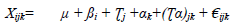Where;

Xijk= any individual observation

µ = Overall mean

ßi = Blocking effect

Ʈj = Effect of varieties

αk = Effect of Poultry Manure

(Ʈα)jk = Interaction effect of Variety and Poultry Manure

ij = experimental error

Stage III: A combined analysis of variance was done for yield measured over four time of planting. Time of planting were considered to have random effects while varieties were considered as fixed effects. The form of analysis of variance showing sources of variation and degrees of freedom is presented in Table 3. The linear additive model for a Combined Analysis of Variance involving Varieties Watermelon, Poultry Manure rates and dates of planting is stated as follows: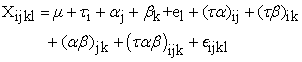Where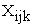= Observation made on the ith variety within the lth replication in the jth Poultry Manure and kth planting date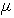= The population or general mean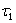= Effect of the ith Variety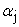= Effect of the jth Poultry Manure rates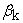= Effect of the kth date of planting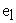= Effect of the lth block/replication within planting date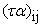= Interaction effect of ith Variety x jth Poultry Manure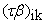= Interaction effect of ith Variety x kth Planting Date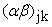= Interaction effect of jth Poultry Manure and kth Planting Date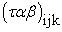= Interaction effect of ith Variety, jth Poultry Manure and kth Planting Date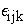= Experimental Error of ith Variety Variation, within jth Poultry Manure, kth Planting Date and lth Block within Date of Planting.

### 4. Experimental Procedures for the Plant Spacing Experiment

The research was carried out at the Teaching and Research Farm of the Department of Crop Production and Landscape Management, Ebonyi State University, Abakaliki. The study region falls within the tropical rain forest zone of South-Eastern Nigeria and it is located between latitude 0604IN and longitude 080 65IE at the elevation of 71.44mm above sea level. It has a bimodal rainfall pattern. It’s rainfall per annum ranges between 1700-2000mm in April to July and August to November for early and late season plantings, respectively. The relative humidity at dry season ranges between 60-80% 32 and the soil belongs to the order ultisols.

Four experiments were laid out as series of similar experiments representing four plant spacings, (0.5m x 0.4m, 0.5m x 0.5m, 1.0m x 0.4m and 1.0m x 0.5m) corresponding to theoretical populations of 50,000, 40,000, 25,000 and 20,000 plants per hectare. The experiments were laid out in a randomized complete block design (RCBD) of three blocks. The total land area for the four plant spacings is 99.2m x 43m while Land cultivation was 8.5m x 22.6m, 8.5m x 27m, 13m x 22.6m and 13m x 27m respectively. Distance between seed beds was 1m for each of the plant spacing while distance between blocks was 1m. The experiment was laid out in three blocks for the four plant spacing. The form of analysis for each plant spacings showing sources of variation and degrees of freedom (General) and (Specific) are shown in Table 5. Figure 1. showed the field layout consisting of three varieties (Kaolack, Koloss F1 and Sugarbaby) of Watermelon and four plant spacings. Seed beds were well prepared by ploughing and harrowing and plots were marked out. Two seeds per hole were sown at the depth of 2cm. Weeds were controlled through manual hoeing and subsequently by hand pulling as the watermelon vines spread and cover the plots to thus suppress weed growth. Poultry Manure rate (5kg/ha) were uniformly applied on all the plots while a combination of Nopest and Snipper pesticides were used to control pest infestation.

• Figure 2. The field layout for Plant Spacing Experiment as Series of Similar Experiments (s1 = Fisrt Plant Spacing and s4 = Fourth Plant Spacing)

Data on yield was collected at maturity. Fruit yield per plot was converted to tones per hectare. The Analysis of Variance was performed using the procedure outlined by 33, 34 for each measured parameter. Means that had a significant F-test were separated using LSD(0.05) 26, 35. The data analysis was carried out in stages.

### 5. Test for Homogeneity of Variance

Bartlett’s 23 test for homogeneity of variance was conducted in order to determine whether or not the assumption of homogeneity was met 10, 35, 36, 37.

Stage I: The data were separately analyzed for yield for each plant spacing, where variances were homogenous (i.e. where the experiments showed no significant Bartlett’s test). This permits the combined analysis of variance for each plant spacing.

Stage II: A combined analysis of variance was done for yield measured for each plant spacing following the procedure of analysis of combined experiments as outlined by 38. The form of combined analysis for each trait measured for plant spacing showing sources of variation and degrees of freedom (General) and (Specific) are shown in Table 4. The linear statistical model used for the analysis of variance is as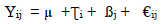Where;

Xij= any individual observation

µ = overall mean

Ʈi= effect of varieties

ßj= blocking effect

ij = experimental error

Stage III: A combined analysis of variance was done for yield measured over four plant spacings. Plant spacings were considered to have random effects while varieties were considered as fixed effects. The form of analysis of variance showing sources of variation and degrees of freedom is presented in Table 5.

The linear additive model for a Combined Analysis of Variance of plant spacings is stated as follows: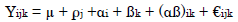Yijk = Observation made on the ith variety within the jth replication in the kth plant spacing

µ = overall experimental mean

ρj = effect of the jth Block/replication within spacing

αi = effect of the ith variety

ßk= effect of the kth plant spacing

(αß)ik = interaction effect of ith variety with the kth plant spacing

ijk = random unit of ith variation within jth block the kth plant spacing on the ith variety component of experimental error.

i = 1, 2 and 3 watermelon varieties

j = 1, 2, 3 and 4 block

k = 1, 2, 3 and 4 plant spacing

### 6. Results

The result shows experiment model analyzed in stages. The example of the result of mean square of the stage I of the analysis of variance and degrees of freedom for yield is presented in Table 3. The example of the results of variance for traits measured from the four plant spacing and the Bartlett’s test for homogeneity of variance is shown in Table 5. The Bartlett’s test was not significant. Based on the non significant Bartlett’s test for homogeneity of variance, the procedure of analysis of combined experiments as outlined by Mclntosh 39 was used to combine the four plant spacing. This is the stage II of the analysis of the experiment model.

The R2 for time of planting experiment model accounted for 65.5% and was observed to be very close to 61.30% gotten by Obi et al., 2009 in his model (using residual analysis to validate rice sowing dates experiment model) while the R2 for plant spacing experiment model is 58.2%. The graphical residual analysis of these two studies which is given by the graph of residuals plotted against predicted values is presented in Figure 3 and Figure 4 below. The plots did not revealed anything particularly troublesome pattern other than a random pattern, although the largest positive residual value observed slightly above 15 stands out from the others. From the graph below, it could be observed that the residuals appear to behave randomly and that suggest that the model fits the data well.

• Figure 3. Plot of Predicted Yield per Plot versus Yield per Plot Residual for Time of Planting Experiment
• Figure 4. Plot of Predicted Yield per plot versus Yield per plot Residual for Plant Spacing Experiment

The question of how to know whether or not the random errors are distributed normally is answered by the normal probability plot. It is used to check whether or not it is reasonable to assume that the random errors inherent in the process have been drawn from a normal distribution. The normal probability is constructed by plotting the sorted values of the residuals against the associated theoretical values of standard normal distribution, distinct curvature or other significant deviations from a straight line as shown below indicate that the random errors are probably not normally distributed. The normal probability plots for these experiment indicate that it is reasonable to assume that the random errors for these processes are drawn from approximately normal distributions. However, since none of the points in the plots for the time of planting and plant spacing deviate much from the linear relationship defined by residuals, it is also reasonable to conclude that there are no outliers in any of these data sets. The normal probability plot is presented in Figure 5 and Figure 6.

• Figure 5. Plot of Residual against Normal scores for Yield per plot for Time of Planting Experiment
• Figure 6. Plot of Residual against Normal scores for Yield per plot for Plant Spacing Experiment

The question of how to know whether or not the random errors are distributed normally is answered by the histogram. The histogram which is more or less a bell-shaped, provides a clearer picture of the shape of the distribution. The bell-shape of the histogram confirms the conclusions from the normal probability plots.

• Figure 7. Histogram of Residual for Yield/plot for Time of Planting Experiment
• Figure 8. Histogram of Residual for Yield/plot for Plant Spacing Experiment

### 7. Discussion

According to Montgomery 40, before the conclusions from the analysis of variance of a design are adopted, the adequacy of the model should be checked. Often the validation of a model seems to consist of nothing more than quoting the R2 (Coeffiecient of Determination) statistic from the fit (which measures the fraction of the total variability in the response that is accounted for by the model). Unfortunately, a high R2 value does not guarantee that the model fits the data well. Use of a model that does not fit the data well cannot provide good answer to the underlying scientific question of how one can know if a model fits the data under investigation. Even though the R2 for both planting dates and plant spacings models accounted for 58.5% and 64.2%, the primary statistical tool for most process modeling application is graphical residual analysis 41. In addition, the normal probability plot also serves as to confirm the adequacy of a model 38, 41.

Different types of plots of the residuals from a fitted model provide information on the adequacy of different aspects of the model. Numerical methods for model validation, such as the R2 statistic, are also useful but usually to a lesser degree than graphical methods. Graphical methods have an advantage over numerical methods for model validation because they readily illustrate a broad range of complex aspects of the relationship between the model and the data. Numerical methods for model validation tend to narrowly focus on a particular aspect of the relationship between the model and the data and often try to compress information into a single descriptive number or test result. If the model’s fit to the data were correct, the residuals would approximate the random errors that make the relationship between the explanatory variables and the response variable a statistical relationship. Therefore, if the residuals appear to behave randomly, it suggests that the model fits the data well. On the other hand, if non-random structure is evident in the residuals, it is a clear sign that the model fits the data poorly. The plot did not revealed anything particularly harmful pattern other than a random pattern, although the largest positive residual value observed slightly above 4 and 10 stands out from the others for both planting dates and plant spacings respectively. It is not enough in the scattered plot to indicate unsuitability of the model for the study. According to 41, it is possible that a particular treatment combination produces slightly more erratic response than the others. The problem moreover is not severe enough to have a negative impact on the analysis and conclusion 41.

The assessment of the sufficiency of the functional part of a model also depends on the scatter plot of the residuals against the predictor variables in the model and against potential predictors that are not included in the model. These are the primary plots used to assess sufficiency of the functional part of the model. Plots in which the residuals do not exhibit any systematic structure indicate that the model fits the data well. Plots of the residuals against other predictor variables, or potential predictors, which exhibit systematic structure, indicate that the form of the function can be improved in some way. In this study, both Figure 3 and Figure 4 did not show a systematic structure.

The question of how to know check whether or not the random errors are distributed normally is answered by the histogram and the normal probability plot. These are used to check whether or not it is reasonable to assume that the random errors inherent in the process have been drawn from a normal distribution. The normality assumption is needed for the error rates we are willing to accept when making decisions about the process. If the random errors are not from a normal distribution, incorrect decisions will be made or less frequency than the stated confidence levels for our inferences indicates.

The normal probability plot is constructed by plotting the sorted values of the residuals against the associated theoretical values from the standard normal distribution. Unlike most residual scatter plots, however, a random scatter of points does not indicate that the assumption being checked is met in this case. Distinct curvature or other significant deviations from a straight line indicate that the random errors are probably not normally distributed. A few points that are far off the line suggest that the data has outliers in it.

The normal probability plot in Figure 5 and Figure 6 indicates that it is reasonable to assume that the random errors for these processes are drawn from approximately normal distributions. In this case, there is a strong linear relationship between the residuals and the theoretical values from the standard normal distribution. Of course the plots do show that the relationship is not perfectly deterministic and it will never be but the linear relationship is still clear. Since none of the points in these plots for both planting dates and plant spacing deviate much from the linear relationship defined by residuals, it is also reasonable to conclude that there are no outliers in any of these data sets.

The graph of residuals plotted against predicted values and the normal probability plot did not reveal anything particularly harmful pattern, although the largest positive residual value observed slightly above 4 and 10 stands out from the others and the normal plot indicated few points at the extreme. These are not enough in the scattered plot to indicate unsuitability of the model for the study. According to 41 it is possible that a particular treatment combination produces slightly more erratic response than the others. The problem more over is not severe enough to have a dramatic impact on the analysis and conclusions 41.

The normal probability plot helps us to determine whether or not it is reasonable to assume that the random errors in a statistical process can be assumed to be drawn from a normal distribution. An advantage of the normal probability plot is that the human eye is very sensitive to deviations from a straight line that might indicate that the errors come from a non-normal distribution. An advantage of the normal probability plot is that the human eye is very sensitive to deviations from a straight line that might indicate that the errors come from a non-normal distribution. However, when the normal probability plot suggests that the normality assumption may not be reasonable, it does not give us a very good idea what the distribution does look like.

A histogram of the residuals from the fit on the other hand, can provide a clearer picture of the shape of the distribution. The fact that the histogram provides more general distributional information than does the normal probability plots suggests that it will be harder to discern deviations from normality than with the more specifically oriented normal probability plot.

The histogram for this studies shown in Figure 7 and Figure 8 showed that the histograms is more or less bell-shaped, confirming the conclusions from the normal probability plots. One important detail to note about normal probability plot and the histogram according to 40 is that they provide information on the distribution of the random errors from the process only if: The functional part of the model is correctly specified, the standard deviation is constant across the data, there is no drift in the process and the random errors are independent from one run to the next.

### 8. Conclusion

A residual analysis procedure was successfully applied to analyze experiment model for plant spacing research on Watermelon using Watermelon yield as a parameter. The procedure proved to be very simple and easy to implement and it can be applied to any statistical model. The residual analysis showed that the conventional experiment model can be adequately used to study the effect of plant spacing on yield of Watermelon in particular and any other annual crop, generally. The plots verify all questions pertaining to model validity. However, the R2 for this plant spacing experiment model was compared with that of 12 experiment and it was discovered that they are similar which further proved Obi Design II to be true 10. The scatter plot, residual plot and the histogram are similar with that of 12.

### References

  Chandra, D. and Mama, G.B. (1988). Effect of planting dates, seedlings age and planting density on late planted wet season rice. – International Rice Research Notes (IRRN) 13: 6-30. In article  Urkurkar, J.S. and Chandrawashi, B.R. (1983). Optimum Transplanting Time for Rice: An Agrometrological Approach.- International Rice Research Notes (IRRN): 8: 6-24. In article  Chand, H. and Singh, R. (1985). Effect of Planting Time on Stem Rot (SR) Incidence, - International Rice Research Notes (IRRN) 10: 6-18. In article  Marzouk, I.A. and El-Bawab, A.M.O. (1999). Effect of sowing date of barley on it’s infestation with the corn leaf Aphid, Ropalosiphum maides (Fitch)(Homoptera Aphididae) and yield components, - Egypt. J. Agric. Res. 77(4): 1493-1499. In article  Soroja, R. and Raju, N. (1983). Influence of planting time on rice leaf folder incidence. - International Rice Research Notes (IRRN): 8: 6-17. In article  Ukwunggwu, M.N. (1984). Planting Time and Stem Borer Incidence in Badeggi, Nigeria.- International Rice Research Notes (IRRN): 9: 1-22. In article View Article  Ukwunggwu, M.N. (1987). Influence of Age of Crop and Time of Planting on Gall Midge (GM) Incidence.- International Rice Research Notes (IRRN): 12: 3-32. In article View Article  Adeoti, A.A. and Emechebe, A.M. (1996). Effect of Sowing Date and Type of Fertilizer on Kenaf In article  Dixon, W.J. (1953). Processing data for outliers.- Biometrica 9(1): 74-79. In article View Article  Obi, I.U. (2006). What have I done as an Agricultural Scientist? (Achievement, Problems and Solution Proposals). – An Inaugural Lecture of the University of Nigeria, Nsukka. Nigeria. Delivered on July 25, 2006. Published by the University of Nigeria Senate Ceremonials Committee. In article  Anyaeze, M.C. (1995). Studies on Time of Planting and on Some Agronomic Characteristics of Sorghum (Sorghum bicolor (L.) Monech) in the Nsukka Plains of Southeastern Nigeria. (Unpublished M.Sc Dessertation – Department of Crop Science. University of Nigeria, Nsukka). In article  Obi, I.U., T. Vange and P.E. Chigbu, 2009. Using residual analysis to validate rice sowing dates experimental model. pp: 153-154. In article View Article  Ogbonna, P.E. (1996). Studies on time of planting and some agronomic characteristics of the local seed type of “Egusi” melon (Cucumis melo) in the derived Savanna Zone of South-Eastern Nigeria (Unpublished M.Sc. Dessertation, - Department of Crop Science, University of Nigeria, Nsukka). In article  Onuoha, E. (1995). Response of maize (Zea mays L.) to time of planting, type and time of fertilizer application in Southeastern Nigeria (unpublished Ph.D Thesis, - Department of Crop Science, University of Nigeria, Nsukka. In article  Swanson, S.P. and Wilhelm, W.W. (1996). Planting date and residue rate effects on growth, partitioning and yield of Corn-Agron. J. 88: 205-210. In article View Article  Pearson, E.S. and Hartley, H.O. (1954). Biometrika Tables for Statisticians. In article View Article  Quesemberry, C.P. and David, H.A. (1961). Some test of outliers.- Biometrika 48(3-4): 379-390. In article View Article  Acikgoz, N. (1987). Effect of Sowing Time and Planting Method on Rice Yield per Day. International Rice Research Notes (IRRN) 12: 1-34. In article  Fakorede, M.A.B., Kim, S.K., Mareck, J.H. and Iken, J.E. (1985). Breeding Strategies and Potentials of available varieties in relation to attaining self-sufficiency in maize production in Nigeria. Presented at the National Symposium “Towards the attainment of Self-Sufficiency of Maize Production in Nigeria 9 March, 1991 I.A.R.& T., Ibadan. In article  Chaudhry, M. (1984). Effect of Sowing Date on Growth and Performance of Six Rice Varieties in Wertern Turkey – IRRN 9: 2-24. In article  Grubbs, F.E. (1969). Procedures for detecting outlying observation in samples.- Technometrics 11(1): 1-21. In article View Article  Stefansky, W. (1972). Rejecting outliers in Factorial Design.- Technometrics 14(2): 469-479. In article View Article  Bartlett, M.S. (1973). Some Examples of Statistical Methods of Research in Agriculture and Applied Biology.- J. Roy. Stat. Soc. Suppl.4:137-185. In article View Article  Levene, H. (1960). In contributions to probability and statistics.- Stanford Univ. press Stanford, Calf., U.S.A. p. 278. In article  Federer, W.T. (1977). A problem in Residual Analysis, An M.S Thesis Problem. Bu-629m.- Biometrics Units Memo. Series Cornell University, Ithaca, New York, U.S.A. In article View Article  Obi, I.U. (2002). Statistical Methods of Detecting Differences between Treatment Means and Research Methodology Issues in Laboratory and Field Experiments. AP Express Publishers, limited, 3 Obollo Road, Nsukka – Nigeria. pp.117 In article  Federer, W.T., Obi, I.U. and Robson, D.S. (1983). Analysis of absolute values of Residuals to Test Distributional Assumption of Linear Model from Balanced Design. Paper No. Bu-22-P, - In the Biometrics Unit, Cornell University, Ithaca, New York, U.S.A. 8pp. In article  Obi, I.U. (2011). Introduction to Regression, Correlation and Covariance Analysis (with worked examples). Printed and bound in Nigeria by Optimal International Ltd., 113 Agbani Rd. Enugu. 2nd Ed. Pp. 105. In article  Shapiro, S.S. and Francia, R.S. (1972). Approximate Analysis of Variance Test for Normality. – Journal of the American Statistical Association 67 (337): 215-223. In article View Article  Chatterjee, S. and Price, B. (1977). Regression Analysis by Example. – New York: John Wiley and Sons, Inc., pp.19-22. In article View Article  Draper, N.R. and Smith, H. (1998): Applied Regression Analysis.- New York. John Wiley and Sons, Inc., 1998. In article View Article  FDALR. (1985). Reconnaissance Soil Survey of Anambra State, Nigeria. Soils Report FDALR. In article  Sanders, D.C., Cure, J.D. & Schultheis, J.R., 1999. Yield response of watermelon to planting density, planting pattern and polyethylene mulch. HortScience 34(7): 1221–1223. In article View Article  Steel, R.G.D. and Torrie, J.H. (1996). Principles and procedures of Statistics: A Biometrical Approach.- Mc Graw-Hill N.Y. 195-233pp. In article  Obi, I.U. (2013b). Post Graduate Lecture Notes on Biometrical Genetics. Department of Crop Production and Landscape Management, Ebonyi State University. Unpublished Memo. In article  Obi, I.U. (2013a). Introduction to Factorial Experiments for Agricultural, Biological and Social Sciences Research. Optimal International Ltd., 113 Agbani Rd. Enugu. 3rd Ed. Pp. 113. In article  Obi, I.U. 2013. Introduction to Factorial Experiment for Agricultural, Biological and Social Science Research (Third Edition). Optimal Computer Solution Ltd, Agbani Road, Enugu, Nigeria VI +47. In article  Maluf, O., Milan, M.T., Spinelli, D. and Martinez, M.E. (2005). Residual analysis applied to S-N data of a surface rolled cast iron – Mat. Res. 8(3) Sao Carlos Jully/Sept. 2005. In article View Article  Mclntosh, M.S. (1983). Analysis of combined experiments.- Agron. J. 75(1): 153-155. In article View Article  Montgomery, D.C. (1991). Design and Analysis of Experiments. Third Edition. – John Willey and Sons, Inc. N.Y. 210-214 pp. In article View Article  NIST/SEMATECH, (2013). NIST/SEMATECH e-handbook of statistics methods. Available, URL: http://www.itl.nist.gov/div898/handbook/eda/section3/eda357.html. In article View Article

Published with license by Science and Education Publishing, Copyright © 2017 I.U. OBI, OKEKE G.C., OSELEBE H.O. and T. VANGEThis work is licensed under a Creative Commons Attribution 4.0 International License. To view a copy of this license, visit http://creativecommons.org/licenses/by/4.0/